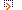# Classical Mechanics DirectoryFeatured Listings

Wave Equation, Wave Packet Solution
Provides some solutions for wave equations.
hyperphysics.phy-astr.gsu.edu/hbase/waves/wavsol.html

Physics Of Sound
Rigorous derivation of sound wave equations from a molecular model of an ideal diatomic gas. General solution of the wave equations. Point source radiating in a moving medium.
www.physicsofsound.com/

Site Listings

Wave Equation, Wave Packet Solution
Provides some solutions for wave equations.
hyperphysics.phy-astr.gsu.edu/hbase/waves/wavsol.html

Physics Of Sound
Rigorous derivation of sound wave equations from a molecular model of an ideal diatomic gas. General solution of the wave equations. Point source radiating in a moving ...
www.physicsofsound.com/

Add Url or Add Site to Submit Site to the Classical Mechanics Directory